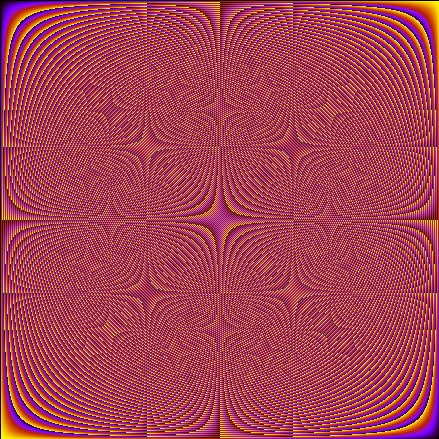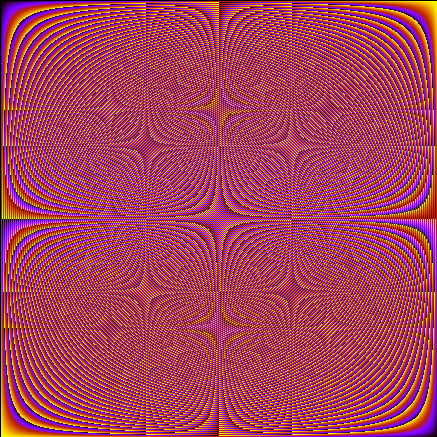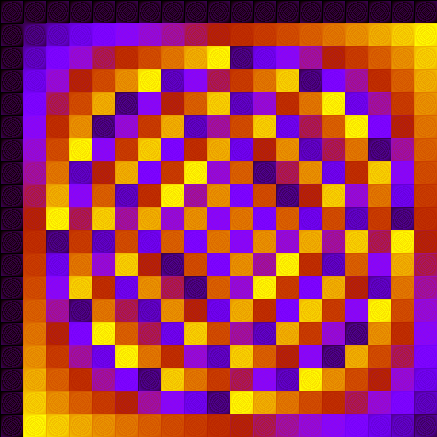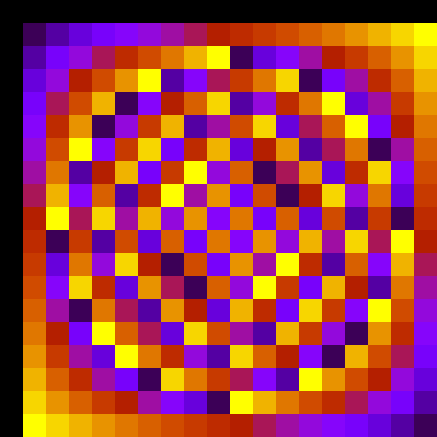# Visualizing group structure, part 2: prime vs. composite, CRT

Some time ago I’ve generated colored addition/multiplication tables in order to visualize group structure. My idea was to compare extension fields of same order with different reduction polynomials, and to compare elliptic curve groups with groups of integers modulo a prime ($\mathbb{Z}_p$). However, as pointed out by an attentive reader, I’ve messed up claiming that the latter group is used by RSA. It is not, it’s used for e.g. DSA, while RSA uses a composite modulus: the product of two prime numbers.

So, how do$\mathbb{Z}_p^*$ and$\mathbb{Z}_n^*$ compare?

### Prime versus composite modulus

Recalling how I’ve generated the tables: I’ve sorted the elements by the default Sage order, and assigned a color for each element according to its position in the list. The$(i,j)$ element of the table is painted with the color assigned to the element that is the result of the multiplication of the i-th and j-th elements.

Here is the table for$\mathbb{Z}_p^*$, for p = 509:And here is the table for$\mathbb{Z}_n^*$, for n = 23*19:As you can see, there isn’t much difference, which is expected: the security of 1024-bit RSA is believed to be roughly the same as 1024-bit DSA, for example.

### Chinese Remainder Theorem

As it is well known, it is possible to speed up RSA using the Chinese Remainder Theorem (CRT) if you know the factorization of$n = pq$. Of course, only the holder of the private key would know that, so only her can employ this optimization. The CRT works by mapping some$x \in \mathbb{Z}_n^*$ to the pair$(x \bmod p, x \bmod q) \in \mathbb{Z}_p^* \times \mathbb{Z}_q^*$, then doing your calculations (e.g. exponentiation) using the pair (adding or multiplicating each element indepently) and then mapping it back to$\mathbb{Z}_n^*$.

Let’s try to visualize the CRT: instead of sorting the elements of$\mathbb{Z}_n^*$ in ascending order, let’s sort using the pairs$(x \bmod p, x \bmod q)$:That’s interesting. I have not enlarged the image, each large block is not uniform. Compare this to$\mathbb{Z}_{19}^*$ (this one is enlarged):It’s the same structure! And if you squint your eyes on the$\mathbb{Z}_n^*$ image, you can see that each large block has some faint structure, which actually is the same as$\mathbb{Z}_{23}^*$. This shows that the CRT decomposes the original group in the two smaller subgroups$\mathbb{Z}_{19}^*$ and$\mathbb{Z}_{23}^*$, which are faster to work with.

1.Guilherme says:
2.妈妈易 says: